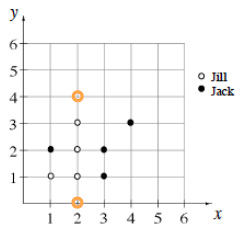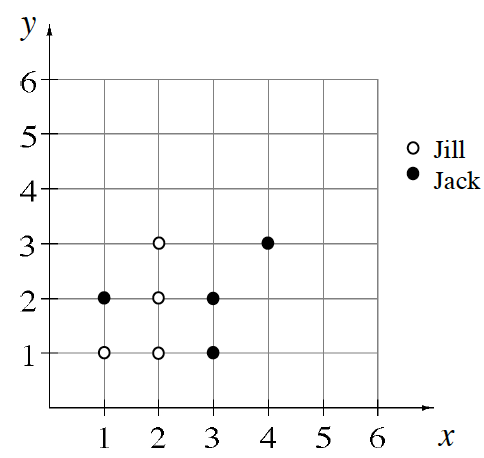### Home > CC1MN > Chapter 5 Unit 5B > Lesson CC1: 5.3.1 > Problem5-73

5-73.

Jack and Jill were each placing points on the grid shown below. Jack’s points are the full circles, and Jill’s are the open circles. Homework Help ✎

1. Record Jack and Jill’s points as ordered pairs.

Remember, in an ordered pair, the x-coordinate (showing the placement along the x-axis) is listed first and the
y-coordinate (showing the placement along the y-axis) is listed second, as in (x, y).

Jill's ordered pairs are $(1,1),(2,1),(2,2)$, and $(2,3)$.
Can you name Jack's ordered pairs?

2. Give the coordinates of one more point that Jill could draw so that she has four of her points in a row.

Can you spot the possible points? Here's a clue: the point should be at the $2$ value on the x-axis, so the ordered pair will look something like this $(2,y)$.

The two possible points are now plotted. Can you record their ordered pairs?